14

7

15

16

# NLSolversBase.jl

Base functionality for optimization and solving systems of equations in Julia.

NLSolversBase.jl is the core, common dependency of several packages in the JuliaNLSolvers family.

# Purpose

The package aims at establishing common ground for Optim.jl, LineSearches.jl, and NLsolve.jl. The common ground is mainly the types used to hold objective related callables, information about the objectives, and an interface to interact with these types.

## NDifferentiable

There are currently three main types: `NonDifferentiable`, `OnceDifferentiable`, and `TwiceDifferentiable`. There's also a more experimental `TwiceDifferentiableHV` for optimization algorithms that use Hessian-vector products. An `NDifferentiable` instance can be used to hold relevant functions for

• Optimization: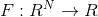• Solving systems of equations: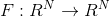The words in front of `Differentiable` in the type names (`Non`, `Once`, `Twice`) are not meant to indicate a specific classification of the function as such (a `OnceDifferentiable` might be constructed for an infinitely differentiable function), but signals to an algorithm if the correct functions have been constructed or if automatic differentiation should be used to further differentiate the function.

## Examples

#### Optimization

Say we want to minimize the Hosaki test function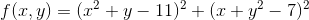The relevant functions are coded in Julia as

``````function f(x)
a = (1.0 - 8.0 * x + 7.0 * x^2 - (7.0 / 3.0) * x^3 + (1.0 / 4.0) * x^4)
return a * x^2 * exp(-x)
end

function g!(G, x)
G = (x^3 - 7.0 * x^2 + 14.0 * x - 8)* x^2 * exp(-x)
G = 2.0 * (1.0 - 8.0 * x + 7.0 * x^2 - (7.0 / 3.0) * x^3 + (1.0 / 4.0) * x^4) * x * exp(-x) - (1.0 - 8.0 * x + 7.0 * x^2 - (7.0 / 3.0) * x^3 + (1.0 / 4.0) * x^4) * x^2 * exp(-x)
end

function fg!(G, x)
g!(G, x)
f(x)
end

function h!(H, x)
H[1, 1] = (3.0 * x^2 - 14.0 * x + 14.0) * x^2 * exp(-x)
H[1, 2] = 2.0 * (x^3 - 7.0 * x^2 + 14.0 * x - 8.0) * x * exp(-x)  - (x^3 - 7.0 * x^2 + 14.0 * x - 8.0) * x^2 * exp(-x)
H[2, 1] =  2.0 * (x^3 - 7.0 * x^2 + 14.0 * x - 8.0) * x * exp(-x)  - (x^3 - 7.0 * x^2 + 14.0 * x - 8.0) * x^2 * exp(-x)
H[2, 2] = 2.0 * (1.0 - 8.0 * x + 7.0 * x^2 - (7.0 / 3.0) * x^3 + (1.0 / 4.0) * x^4) * exp(-x) - 4.0 * ( 1.0 - 8.0 * x + 7.0 *  x^2 - (7.0 / 3.0) * x^3 + (1.0 / 4.0) * x^4) * x * exp(-x) + (1.0 - 8.0 * x + 7.0 * x^2 - (7.0 / 3.0) * x^3 + (1.0 / 4.0) * x^4) * x^2 * exp(-x)
end
``````

The `NDifferentiable` interface can be used as shown below to create various objectives:

``````x = zeros(2)
nd   = NonDifferentiable(f, x)
od   = OnceDifferentiable(f, g!, x)
odfg = OnceDifferentiable(f, g!, fg! x)
td1  = Twicedifferentiable(f, g!, h! x)
tdfg = Twicedifferentiable(f, g!, fg!, h! x)
``````

#### Multivalued objective

If we consider the gradient of the Himmelblau function above, we can try to solve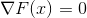without caring about the objective value. Then we can still create `NDifferentiable`s, but we need to specify the cache to hold the value of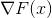. Currently, the only relevant ones are `NonDifferentiable` and `OnceDifferentiable`. `TwiceDifferentiable` could be used for higher order (tensor) methods, though they are rarely worth the cost. The relevant functions coded in Julia are:

``````function f!(F, x)
F = (x^3 - 7.0 * x^2 + 14.0 * x - 8)* x^2 * exp(-x)
F = 2.0 * (1.0 - 8.0 * x + 7.0 * x^2 - (7.0 / 3.0) * x^3 + (1.0 / 4.0) * x^4) * x * exp(-x) - (1.0 - 8.0 * x + 7.0 * x^2 - (7.0 / 3.0) * x^3 + (1.0 / 4.0) * x^4) * x^2 * exp(-x)
end

function j!(J, x)
J[1, 1] = (3.0 * x^2 - 14.0 * x + 14.0) * x^2 * exp(-x)
J[1, 2] = 2.0 * (x^3 - 7.0 * x^2 + 14.0 * x - 8.0) * x * exp(-x)  - (x^3 - 7.0 * x^2 + 14.0 * x - 8.0) * x^2 * exp(-x)
J[2, 1] =  2.0 * (x^3 - 7.0 * x^2 + 14.0 * x - 8.0) * x * exp(-x)  - (x^3 - 7.0 * x^2 + 14.0 * x - 8.0) * x^2 * exp(-x)
J[2, 2] = 2.0 * (1.0 - 8.0 * x + 7.0 * x^2 - (7.0 / 3.0) * x^3 + (1.0 / 4.0) * x^4) * exp(-x) - 4.0 * ( 1.0 - 8.0 * x + 7.0 *  x^2 - (7.0 / 3.0) * x^3 + (1.0 / 4.0) * x^4) * x * exp(-x) + (1.0 - 8.0 * x + 7.0 * x^2 - (7.0 / 3.0) * x^3 + (1.0 / 4.0) * x^4) * x^2 * exp(-x)
end

function fj!(F, G, x)
g!(G, x)
f!(F, x)
end
``````

The `NDifferentiable` interface can be used as shown below to create various objectives:

``````x = zeros(2)
F = zeros(2)
nd   = NonDifferentiable(f!, x, F)
od   = OnceDifferentiable(f!, j!, x, F)
odfj = OnceDifferentiable(f!, j!, fj! x, F)
``````

## Interface

To extract information about the objective, and to update given some input, we provide a function based interface. For all purposes it should be possible to use a function to extract/update information, and no field access should be necessary. Actually, we proactively discourage it, as it makes it much more difficult to make changes in the future.

### Single-valued objectives

To retrieve relevant information about single-valued functions, the following functions are available where applicable:

``````# obj is the objective function defined as shown above
value(df)       # return the objective evaluated at df.x_f
hessian(df)     # return the hessian evaluated at df.x_h
``````

To update the various quantities, use:

``````# obj is the objective function defined as shown above
value!(df, x)     # update the objective if !(df.x_f==x) and set df.x_f to x
value!!(df, x)    # update the objective and set df.x_f to x
gradient!(df, x)  # update the gradient if !(df.x_df==x) and set df.x_df to x
hessian!(df,x)    # update the hessian if !(df.x_df==x) and set df.x_h to x
hessian!!(df,x)   # update the hessian and set df.x_h to x
``````

### Multivalued

To retrieve relevant information about multivalued functions, the following functions are available where applicable:

``````# obj is the objective function defined as shown above
value(df)    # return the objective evaluated at df.x_f
jacobian(df) # return the jacobian evaluated at df.x_df
jacobian(df) # return the jacobian evaluated at df.x_df
``````

To update the various quantities, use:

``````# obj is the objective function defined as shown above
value!(df, x)     # update the objective if !(df.x_f==x) and set df.x_f to x
value!!(df, x)    # update the objective and set df.x_f to x
jacobian!(df, x)  # update the jacobian if !(df.x_df==x) and set df.x_df to x
jacobian!!(df, x) # update the jacobian and set df.x_df to x
``````

## Special single-function interface

In some cases the objective and partial derivaties share common terms that are expensive to calculate. One such case is if the underlying problem requires solution of a model or simulation of a some system. In that case the `only_fg!`/`only_fj!` and `only_fgh!` interfaces can be used.

### Example

Say we have some common functionality in `common_calc(...)` that is used in both the objective and partial derivative. Then we might construct a OnceDifferentiable instance as

``````function f(x)
common_calc(...)
# calculations specific to f
return f
end
function g!(G, x)
common_calc(...)
# mutating calculations specific to g!
end
OnceDifferentiable(f, g!, x0)
``````

However, in many algorithms `f` and `g!` are evaluated together, so the common calculations are done twice instead of once. We can use the special interface as shown below.

``````function fg!(F, G, x)
common_calc(...)
if !(G == nothing)
# mutating calculations specific to g!
end
if !(F == nothing)
# calculations specific to f
return f
end
end
OnceDifferentiable(only_fg!(fg!), x0)
``````

Notice the important check in the `if` statements. This makes sure that `G` is only updated when we want to, and, if only `G` is to be updated, that we don't calculate the objective.

03/11/2017

2 months ago

95 commits